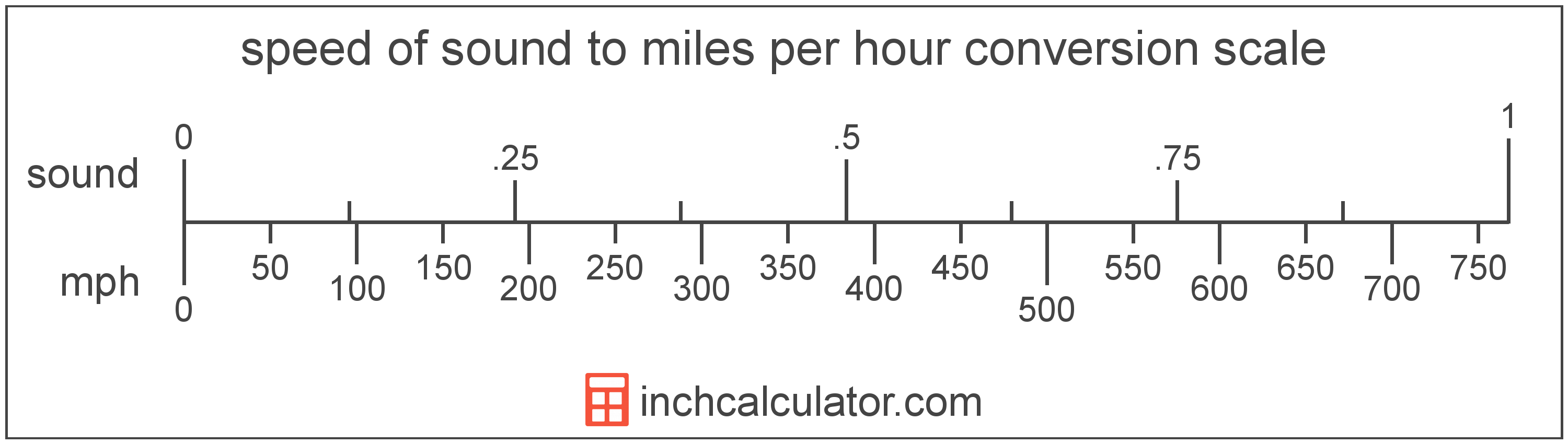# Miles per Hour to Speed of Sound Converter

Enter the speed in miles per hour below to get the value converted to speed of sound.

Results in Speed of Sound:1 mph = 0.001303 sound

Do you want to convert speed of sound to miles per hour?

## How to Convert Miles per Hour to Speed of Sound

To convert a measurement in miles per hour to a measurement in speed of sound, multiply the speed by the following conversion ratio: 0.001303 speed of sound/mile per hour.

Since one mile per hour is equal to 0.001303 speed of sound, you can use this simple formula to convert:

speed of sound = miles per hour × 0.001303

The speed in speed of sound is equal to the speed in miles per hour multiplied by 0.001303.

For example, here's how to convert 500 miles per hour to speed of sound using the formula above.
speed of sound = (500 mph × 0.001303) = 0.651662 soundMiles per hour and speed of sound are both units used to measure speed. Keep reading to learn more about each unit of measure.

## What Are Miles per Hour?

Miles per hour are a measurement of speed expressing the distance traveled in miles in one hour.

The mile per hour is a US customary and imperial unit of speed. Miles per hour can be abbreviated as mph, and are also sometimes abbreviated as mi/h or MPH. For example, 1 mile per hour can be written as 1 mph, 1 mi/h, or 1 MPH.

Miles per hour can be expressed using the formula:
vmph = dmi / thr

The velocity in miles per hour is equal to the distance in miles divided by time in hours.

## What Is the Speed of Sound?

The speed of sound is the distance a sound wave travels through an elastic medium. The speed of sound through air at 20 °C is equal to 343 meters per second, or roughly 767 miles per hour.

Speed of sound can be abbreviated as sound; for example, 1 speed of sound can be written as 1 sound.

## Mile per Hour to Speed of Sound Conversion Table

Table showing various mile per hour measurements converted to speed of sound.
Miles Per Hour Speed Of Sound
1 mph 0.001303 sound
2 mph 0.002607 sound
3 mph 0.00391 sound
4 mph 0.005213 sound
5 mph 0.006517 sound
6 mph 0.00782 sound
7 mph 0.009123 sound
8 mph 0.010427 sound
9 mph 0.01173 sound
10 mph 0.013033 sound
20 mph 0.026066 sound
30 mph 0.0391 sound
40 mph 0.052133 sound
50 mph 0.065166 sound
60 mph 0.078199 sound
70 mph 0.091233 sound
80 mph 0.104266 sound
90 mph 0.117299 sound
100 mph 0.130332 sound
200 mph 0.260665 sound
300 mph 0.390997 sound
400 mph 0.521329 sound
500 mph 0.651662 sound
600 mph 0.781994 sound
700 mph 0.912327 sound
800 mph 1.0427 sound
900 mph 1.173 sound
1,000 mph 1.3033 sound

## References

1. Wikipedia, Miles per hour, https://en.wikipedia.org/wiki/Miles_per_hour
2. CK-12, Speed of Sound, https://www.ck12.org/physics/speed-of-sound/lesson/Speed-of-Sound-MS-PS/